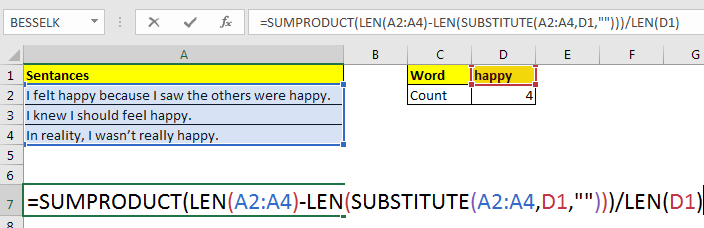How to Count Occurrences of a Word in an Excel Range in Excel

We have already learnt how to count cells that contain a specific text using COUNTIF function. In this article, we will learn how to count how many times a word appears in excel range. In other words, we will count how many times a word occurred in an excel range.Generic Formula

=SUMPRODUCT(LEN(range)-LEN(SUBSTITUTE(range,word,"")))/LEN(word)

Range: The range in which you are trying to count the specific word.
Word: The word you want to count.

Let’s take an example and understand how it works.

Example: Count “happy” word in excel range.
Here we have some sentences in different cells. We need to count the occurrences of word “happy” in that range.

Write this formula in cell D2.

=SUMPRODUCT(LEN(A2:A4)-LEN(< a href="https://www.exceltip.com/excel-text-formulas/excel-substitute-function.html">SUBSTITUTE(A2:A4,D1,"")))/LEN(D1)

Using this function excel counts how many times the specific word “happy” appears in range A2:A4. This excel formula returns the count as 4.
How it works?
The idea is to get sum of character count of given word in range and then divide by the length of the word. For example if Happy is 4 times occurring in a range, it’s total length 20 (4*5) in range. If we divide 20 by 5 we get 4. Which is the count of word in range.
Let’s tear it down from inside.
LEN(A2:A4): this returns count of characters in each cell as an array {49;27;34}.

Next LEN(SUBSTITUTE(A2:A4,D1,"")): The substitute function repaces word in D1 with “” in each cell of range A2:A4. Then Len function returns count of characters from this substituted sentences in an array {39;22;29}.

LEN(D1): this returns the length of word in D1 which 5 (happy).

Now the formula is simplified to SUMPRODUCT({49;27;34} - {39;22;29})/5. After subtraction of arrays, SUMPRODUCT has SUMPRODUCT({10;5;5})/5. The function adds the array and we get 20/5. Which gives us our result 4.

Counting Case-Insensitive
Since SUBSTITUTE is case sensitive, above formula will ignore any word not having same case, i.e. “Happy”. To make the above formula ignore case, we must change case of each word to case of word we are looking for. This how it’s done.

=SUMPRODUCT(LEN(A2:A4)-LEN(SUBSTITUTE(UPPER(A2:A4),UPPER(D1),"")))/LEN(D1)

Now the case doesn’t matter anymore. This function will count each word in D1 irrespective of case.

Possible Errors:
Word part of another word will be counted: In this example, if we had word “happyness” (just for example, I know there’s no word as this) it would have been counted too. To avoid this you could have surrounded the words with speces, “ ” &D1& “ ”. But when word appears first or last in sentence, this will fail too.

Popular Articles

50 Excel Shortcut to Increase Your Productivity: Get faster at your task. These 50 shortcuts will make you work even faster on Excel.

How to use the VLOOKUP Function in Excel: This is one of the most used and popular functions of excel that is used to lookup value from different ranges and sheets.

How to use the COUNTIF function in Excel: Count values with conditions using this amazing function. You don't need to filter your data to count specific values. Countif function is essential to prepare your dashboard.

How to use the SUMIF Function in Excel: This is another dashboard essential function. This helps you sum up values on specific conditions.

Terms and Conditions of use

The applications/code on this site are distributed as is and without warranties or liability. In no event shall the owner of the copyrights, or the authors of the applications/code be liable for any loss of profit, any problems or any damage resulting from the use or evaluation of the applications/code.